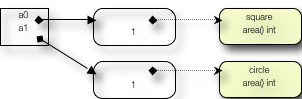# Go 语言 Interface 漫谈

Go 语言的诞生，是三个有很强个性的设计师共同完成的。Go 语言的定位，就象三维坐标系中的一个点，在强类型、动态和并发这三个特性维度上，分别代表了 Ken、Robert 和 Rob 三人的创造思维的投影。

   i := 0
j := 0i

Go 的面向对象不支持重载也只有有限的继承。目的很明确，Go 是要简化类型系统、尤其是已经被过度复杂化了的面向对象的类的类型系统。

package main

import "fmt"

type square struct{ r int }
type circle struct{ r int }

func (s square) area() int { return s.r * s.r }
func (c circle) area() int { return c.r * 3 }

func main() {
s := square{1}
c := circle{1}
fmt.Println(s, c, s.area()+c.area())
}

package main

import "fmt"

type square struct{ r int }
type circle struct{ r int }

func (s square) area() int { return s.r * s.r }
func (c circle) area() int { return c.r * 3 }

func main() {
s := square{1}
c := circle{1}
a := interface{}{s, c}
fmt.Println(s, c, a)

sum := 0
for _, t := range a {
switch v := t.(type) {
case square:
sum += v.area()
case circle:
sum += v.area()
}
}
fmt.Println(sum)
}

range 是 Go 的遍历语句，此处的变量 t 被依次赋值为数组 a 的单元值，它们还都是空界面类型，所以我们只需用 switch 测试并转换成具体类型的变量 v，就可以使用这个具体类型所定义的 area 方法，得到相应的面积，并进行求和运算了。type Err struct {}
func (_ *Err) Error() string {
return "To err is human"
}


func NoErr(ok bool) error {
if !ok {
return &Err{}
}
return nil
}

func main() {
fmt.Println(NoErr(true))
fmt.Println(NoErr(false))

// Output:
//           // To err is human  } 

func ToErr(ok bool) error {
var e *Err = nil
if ok {
e = &Err{}
}
return e
}

func main() {
fmt.Println(ToErr(true) == nil)  //false
fmt.Println(ToErr(false) == nil)  //false
}